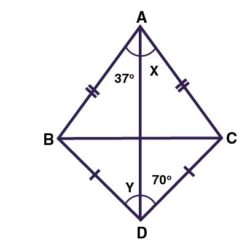# Calculate the angle x and angle y value in the given figure?From the given figure

AB = AC and BD = CD

Then the diagonally opposite angles are equal.

∠ABD = ∠ACD Or ∠BDC = ∠BAC

y + 70 = x + 37

The x = 70 and y = 37